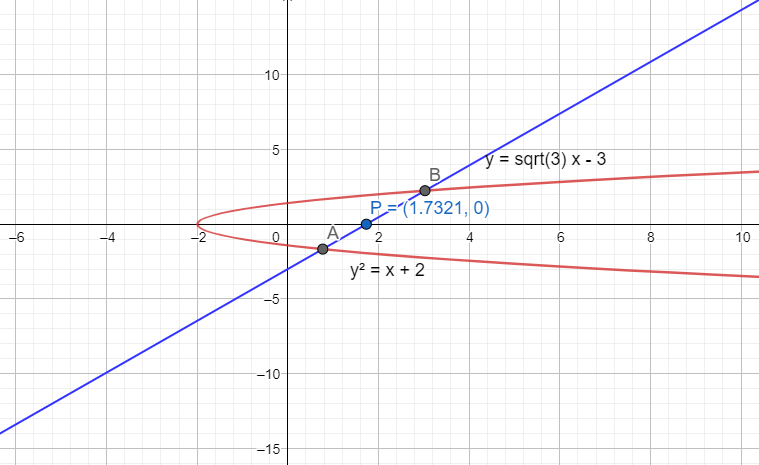Courses
Courses for Kids
Free study material
Free LIVE classes
MoreLIVE
Join Vedantu’s FREE Mastercalss

# If the line $y-\sqrt{3}x+3=0$ cuts the parabola ${{y}^{2}}=x+2$ at $A$ and $B$, then$PA.PB$is equal to [where $P=\left( \sqrt{3},0 \right)$](a) $\dfrac{4\left( \sqrt{3}+2 \right)}{3}$(b) $\dfrac{4\left( 2-\sqrt{3} \right)}{3}$(c) $\dfrac{4\sqrt{3}}{2}$(d) $\dfrac{2\left( \sqrt{3}+2 \right)}{3}$Verified
360.9k+ views
Hint: The parametric form of the equation of straight line, $\dfrac{x-{{x}_{1}}}{\cos \theta }=\dfrac{y-{{y}_{1}}}{\sin \theta }=r$ is used in this question.

The line given in the question is $y-\sqrt{3}x+3=0$ and the equation of the parabola is given as ${{y}^{2}}=x+2$.
It is said in the question that the line cuts the parabola at points $A$ and $B$. A point $P$ with coordinates $\left( \sqrt{3},0 \right)$ is also given. So, we can plot the graph with all the details as shown below,We need to find the values of $PA$ and $PB$ to solve the question. $PA$ and $PB$ represent the distance of the line joined by the points $P,A$ and $P,B$ respectively.
The parametric form of a straight line passing through the point $\left( {{x}_{1}},{{y}_{1}} \right)$ and making an angle of $\theta$ with the positive direction of the x-axis is given by,
$\dfrac{x-{{x}_{1}}}{\cos \theta }=\dfrac{y-{{y}_{1}}}{\sin \theta }=r\ldots \ldots \ldots (i)$
where $r$ is the distance between the two points with coordinates $\left( x,y \right)$ and $\left( {{x}_{1}},{{y}_{1}} \right)$.
In this question, $PA$ and $PB$ are equivalent to the distance $r$ mentioned above.
We have the coordinates of the point $\left( {{x}_{1}},{{y}_{1}} \right)=\left( \sqrt{3},0 \right)$. So, the first step is to find the angle $\theta$.
For that, we need to consider the equation of the line and rearrange it in the slope-intercept form,
$y-\sqrt{3}x+3=0$
$y=\sqrt{3}x-3$
Comparing it with the slope-intercept form given by $y=mx+c$, we get the slope as $m=\sqrt{3}=\tan \theta$.
The angle $\theta$ can hence be computed as below,
\begin{align} & \tan \theta =\sqrt{3} \\ & \theta ={{\tan }^{-1}}\sqrt{3} \\ & \theta =60{}^\circ \\ \end{align}
Now substituting the obtained results in the equation $(i)$,
$\dfrac{x-\sqrt{3}}{\cos 60{}^\circ }=\dfrac{y-0}{\sin 60{}^\circ }=r$
Substituting the values of $\cos 60{}^\circ =\dfrac{1}{2},\sin 60{}^\circ =\dfrac{\sqrt{3}}{2}$, we get,
$\dfrac{x-\sqrt{3}}{\dfrac{1}{2}}=\dfrac{y-0}{\dfrac{\sqrt{3}}{2}}=r$
Equating both $x$ and $y$ to $r$,
$\dfrac{x-\sqrt{3}}{\dfrac{1}{2}}=r,\dfrac{y-0}{\dfrac{\sqrt{3}}{2}}=r$
\begin{align} & x-\sqrt{3}=\dfrac{r}{2},y=\dfrac{\sqrt{3}}{2}r \\ & x=\sqrt{3}+\dfrac{r}{2},y=\dfrac{\sqrt{3}}{2}r \\ \end{align}
So, we have the coordinates of the point, $A$ or $B$ as $\left( \sqrt{3}+\dfrac{r}{2},\dfrac{\sqrt{3}}{2}r \right)$.
As per the question, we know that this point cuts the parabola ${{y}^{2}}=x+2$, so it can be substituted in the equation for the parabola as,
\begin{align} & {{\left( \dfrac{\sqrt{3}}{2}r \right)}^{2}}=\left( \sqrt{3}+\dfrac{r}{2} \right)+2 \\ & \dfrac{3{{r}^{2}}}{4}=\dfrac{r}{2}+\left( \sqrt{3}+2 \right) \\ & \dfrac{3{{r}^{2}}}{4}-\dfrac{r}{2}-\left( \sqrt{3}+2 \right)=0 \\ \end{align}
This is in the form of a quadratic equation in $r$, so we can get the values of $r$ using the formula as,
\begin{align} & r=\dfrac{-b\pm \sqrt{{{b}^{2}}-4ac}}{2a} \\ & r=\dfrac{-\left( -\dfrac{1}{2} \right)\pm \sqrt{{{\left( -\dfrac{1}{2} \right)}^{2}}-4\times \left( \dfrac{3}{4} \right)\times -\left( \sqrt{3}+2 \right)}}{2\left( \dfrac{3}{4} \right)} \\ & r=\dfrac{\dfrac{1}{2}\pm \sqrt{\dfrac{1}{4}+\left( \dfrac{12}{4} \right)\left( \sqrt{3}+2 \right)}}{\left( \dfrac{3}{2} \right)} \\ \end{align}
Taking $\dfrac{1}{4}$ outside from the root,
\begin{align} & r=\dfrac{\dfrac{1}{2}\pm \sqrt{\dfrac{1}{4}\left( 1+12\left( \sqrt{3}+2 \right) \right)}}{\left( \dfrac{3}{2} \right)} \\ & r=\dfrac{\dfrac{1}{2}\pm \dfrac{1}{2}\sqrt{1+12\left( \sqrt{3}+2 \right)}}{\left( \dfrac{3}{2} \right)} \\ \end{align}
Cancelling out $\dfrac{1}{2}$,
$r=\dfrac{1\pm \sqrt{1+12\left( \sqrt{3}+2 \right)}}{3}$
Therefore, we have the roots as,
\begin{align} & PA=\dfrac{1+\sqrt{1+12\left( \sqrt{3}+2 \right)}}{3} \\ & PB=\dfrac{1-\sqrt{1+12\left( \sqrt{3}+2 \right)}}{3} \\ \end{align}
Now, we can compute $PA.PB$ as,
$PA.PB=\dfrac{1+\sqrt{1+12\left( \sqrt{3}+2 \right)}}{3}\times \dfrac{1-\sqrt{1+12\left( \sqrt{3}+2 \right)}}{3}$
Applying the identity $\left( x+y \right)\left( x-y \right)={{x}^{2}}-{{y}^{2}}$,
\begin{align} & PA.PB=\dfrac{1}{9}\times \left[ {{1}^{2}}-{{\sqrt{1+12\left( \sqrt{3}+2 \right)}}^{2}} \right] \\ & PA.PB=\dfrac{1}{9}\times \left[ 1-1-12\left( \sqrt{3}+2 \right) \right] \\ & PA.PB=\dfrac{1}{9}\times \left[ -12\left( \sqrt{3}+2 \right) \right] \\ & PA.PB=\dfrac{-12}{9}\times \left( \sqrt{3}+2 \right) \\ & PA.PB=\dfrac{-4}{3}\times \left( \sqrt{3}+2 \right) \\ \end{align}
We have to consider the modulus for the distance, so we get the value of $PA.PB$ as $\left| \dfrac{-4\left( \sqrt{3}+2 \right)}{3} \right|=\dfrac{4\left( \sqrt{3}+2 \right)}{3}$.
Hence option (a) is the correct answer.

Note: The last portion of the solution can be done more easily by using the fact that the product of the roots of a quadratic equation $a{{x}^{2}}+bx+c=0$ can be obtained as $\dfrac{c}{a}$. So, for this question,$\dfrac{3{{r}^{2}}}{4}-\dfrac{r}{2}-\left( \sqrt{3}+2 \right)=0$, the product of the roots $PA$ and $PB$ can be obtained as \begin{align} & PA.PB=\dfrac{-\left( \sqrt{3}+2 \right)}{\dfrac{3}{4}} \\ & PA.PB=\dfrac{-4\left( \sqrt{3}+2 \right)}{3} \\ & PA.PB=\left| \dfrac{-4\left( \sqrt{3}+2 \right)}{3} \right|\Rightarrow \dfrac{4\left( \sqrt{3}+2 \right)}{3} \\ \end{align}
Last updated date: 21st Sep 2023
Total views: 360.9k
Views today: 4.60k